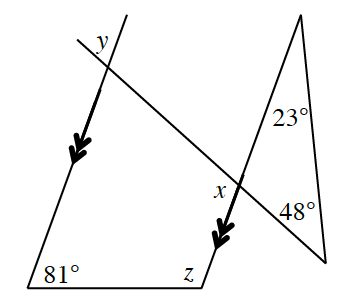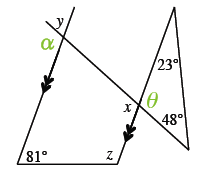### Home > GC > Chapter 10 > Lesson 10.2.2 > Problem10-71

10-71.Examine the diagram at right. Use the given geometric relationships to solve for $x$, $y$, and $z$. Be sure to justify your work by stating the geometric relationship and applicable theorem.

Use the Triangle Angle Sum Theorem to find $x$.

$23°+48°+θ=180°$
$θ=109°$Vertical angles are congruent. Use this information to find $x$.

$α+y=180°$
When solving for $α$, remember that parallel lines have congruent corresponding angles.

$y=71°$
$x=109°$